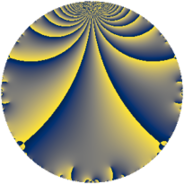# Properties

 Label 3025.2.a.aLevel $3025$ Weight $2$ Character orbit 3025.a Self dual yes Analytic conductor $24.155$ Analytic rank $0$ Dimension $1$ CM no Inner twists $1$

# Related objects

## Newspace parameters

 Level: $$N$$ $$=$$ $$3025 = 5^{2} \cdot 11^{2}$$ Weight: $$k$$ $$=$$ $$2$$ Character orbit: $$[\chi]$$ $$=$$ 3025.a (trivial)

## Newform invariants

 Self dual: yes Analytic conductor: $$24.1547466114$$ Analytic rank: $$0$$ Dimension: $$1$$ Coefficient field: $$\mathbb{Q}$$ Coefficient ring: $$\mathbb{Z}$$ Coefficient ring index: $$1$$ Twist minimal: no (minimal twist has level 11) Fricke sign: $$-1$$ Sato-Tate group: $\mathrm{SU}(2)$

## $q$-expansion

 $$f(q)$$ $$=$$ $$q - 2q^{2} + q^{3} + 2q^{4} - 2q^{6} - 2q^{7} - 2q^{9} + O(q^{10})$$ $$q - 2q^{2} + q^{3} + 2q^{4} - 2q^{6} - 2q^{7} - 2q^{9} + 2q^{12} + 4q^{13} + 4q^{14} - 4q^{16} - 2q^{17} + 4q^{18} - 2q^{21} + q^{23} - 8q^{26} - 5q^{27} - 4q^{28} + 7q^{31} + 8q^{32} + 4q^{34} - 4q^{36} - 3q^{37} + 4q^{39} + 8q^{41} + 4q^{42} - 6q^{43} - 2q^{46} - 8q^{47} - 4q^{48} - 3q^{49} - 2q^{51} + 8q^{52} + 6q^{53} + 10q^{54} + 5q^{59} - 12q^{61} - 14q^{62} + 4q^{63} - 8q^{64} + 7q^{67} - 4q^{68} + q^{69} - 3q^{71} + 4q^{73} + 6q^{74} - 8q^{78} + 10q^{79} + q^{81} - 16q^{82} - 6q^{83} - 4q^{84} + 12q^{86} + 15q^{89} - 8q^{91} + 2q^{92} + 7q^{93} + 16q^{94} + 8q^{96} + 7q^{97} + 6q^{98} + O(q^{100})$$

## Embeddings

For each embedding $$\iota_m$$ of the coefficient field, the values $$\iota_m(a_n)$$ are shown below.

For more information on an embedded modular form you can click on its label.

Label $$\iota_m(\nu)$$ $$a_{2}$$ $$a_{3}$$ $$a_{4}$$ $$a_{5}$$ $$a_{6}$$ $$a_{7}$$ $$a_{8}$$ $$a_{9}$$ $$a_{10}$$
1.1
 0
−2.00000 1.00000 2.00000 0 −2.00000 −2.00000 0 −2.00000 0
 $$n$$: e.g. 2-40 or 990-1000 Significant digits: Format: Complex embeddings Normalized embeddings Satake parameters Satake angles

## Atkin-Lehner signs

$$p$$ Sign
$$5$$ $$1$$
$$11$$ $$-1$$

## Inner twists

This newform does not admit any (nontrivial) inner twists.

## Twists

By twisting character orbit
Char Parity Ord Mult Type Twist Min Dim
1.a even 1 1 trivial 3025.2.a.a 1
5.b even 2 1 121.2.a.d 1
11.b odd 2 1 275.2.a.b 1
15.d odd 2 1 1089.2.a.b 1
20.d odd 2 1 1936.2.a.i 1
33.d even 2 1 2475.2.a.a 1
35.c odd 2 1 5929.2.a.h 1
40.e odd 2 1 7744.2.a.k 1
40.f even 2 1 7744.2.a.x 1
44.c even 2 1 4400.2.a.i 1
55.d odd 2 1 11.2.a.a 1
55.e even 4 2 275.2.b.a 2
55.h odd 10 4 121.2.c.e 4
55.j even 10 4 121.2.c.a 4
165.d even 2 1 99.2.a.d 1
165.l odd 4 2 2475.2.c.a 2
220.g even 2 1 176.2.a.b 1
220.i odd 4 2 4400.2.b.h 2
385.h even 2 1 539.2.a.a 1
385.o even 6 2 539.2.e.g 2
385.q odd 6 2 539.2.e.h 2
440.c even 2 1 704.2.a.c 1
440.o odd 2 1 704.2.a.h 1
495.o odd 6 2 891.2.e.k 2
495.r even 6 2 891.2.e.b 2
660.g odd 2 1 1584.2.a.g 1
715.c odd 2 1 1859.2.a.b 1
880.x odd 4 2 2816.2.c.j 2
880.bi even 4 2 2816.2.c.f 2
935.h odd 2 1 3179.2.a.a 1
1045.e even 2 1 3971.2.a.b 1
1155.e odd 2 1 4851.2.a.t 1
1265.f even 2 1 5819.2.a.a 1
1320.b odd 2 1 6336.2.a.bu 1
1320.u even 2 1 6336.2.a.br 1
1540.b odd 2 1 8624.2.a.j 1
1595.e odd 2 1 9251.2.a.d 1

By twisted newform orbit
Twist Min Dim Char Parity Ord Mult Type
11.2.a.a 1 55.d odd 2 1
99.2.a.d 1 165.d even 2 1
121.2.a.d 1 5.b even 2 1
121.2.c.a 4 55.j even 10 4
121.2.c.e 4 55.h odd 10 4
176.2.a.b 1 220.g even 2 1
275.2.a.b 1 11.b odd 2 1
275.2.b.a 2 55.e even 4 2
539.2.a.a 1 385.h even 2 1
539.2.e.g 2 385.o even 6 2
539.2.e.h 2 385.q odd 6 2
704.2.a.c 1 440.c even 2 1
704.2.a.h 1 440.o odd 2 1
891.2.e.b 2 495.r even 6 2
891.2.e.k 2 495.o odd 6 2
1089.2.a.b 1 15.d odd 2 1
1584.2.a.g 1 660.g odd 2 1
1859.2.a.b 1 715.c odd 2 1
1936.2.a.i 1 20.d odd 2 1
2475.2.a.a 1 33.d even 2 1
2475.2.c.a 2 165.l odd 4 2
2816.2.c.f 2 880.bi even 4 2
2816.2.c.j 2 880.x odd 4 2
3025.2.a.a 1 1.a even 1 1 trivial
3179.2.a.a 1 935.h odd 2 1
3971.2.a.b 1 1045.e even 2 1
4400.2.a.i 1 44.c even 2 1
4400.2.b.h 2 220.i odd 4 2
4851.2.a.t 1 1155.e odd 2 1
5819.2.a.a 1 1265.f even 2 1
5929.2.a.h 1 35.c odd 2 1
6336.2.a.br 1 1320.u even 2 1
6336.2.a.bu 1 1320.b odd 2 1
7744.2.a.k 1 40.e odd 2 1
7744.2.a.x 1 40.f even 2 1
8624.2.a.j 1 1540.b odd 2 1
9251.2.a.d 1 1595.e odd 2 1

## Hecke kernels

This newform subspace can be constructed as the intersection of the kernels of the following linear operators acting on $$S_{2}^{\mathrm{new}}(\Gamma_0(3025))$$:

 $$T_{2} + 2$$ $$T_{3} - 1$$ $$T_{19}$$

## Hecke characteristic polynomials

$p$ $F_p(T)$
$2$ $$2 + T$$
$3$ $$-1 + T$$
$5$ $$T$$
$7$ $$2 + T$$
$11$ $$T$$
$13$ $$-4 + T$$
$17$ $$2 + T$$
$19$ $$T$$
$23$ $$-1 + T$$
$29$ $$T$$
$31$ $$-7 + T$$
$37$ $$3 + T$$
$41$ $$-8 + T$$
$43$ $$6 + T$$
$47$ $$8 + T$$
$53$ $$-6 + T$$
$59$ $$-5 + T$$
$61$ $$12 + T$$
$67$ $$-7 + T$$
$71$ $$3 + T$$
$73$ $$-4 + T$$
$79$ $$-10 + T$$
$83$ $$6 + T$$
$89$ $$-15 + T$$
$97$ $$-7 + T$$